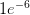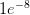# Regressions with Multiple Fixed Effects – Comparing Stata and R

In my paper on the impact of the recent fracking boom on local economic outcomes, I am estimating models with multiple fixed effects. These fixed effects are useful, because they take out, e.g. industry specific heterogeneity at the county level – or state specific time shocks.

The models can take the form:

$y_{cist} = \alpha_{ci} + b_{st} + \gamma_{it}+ X_{cist}'\beta + \epsilon_{cist}$

where $\alpha_{ci}$ is a set of county-industry, $b_{ci}$ a set of state-time and $\gamma_{it}$ is a set of industry-time fixed effects.

Such a specification takes out arbitrary state-specific time shocks and industry specific time shocks, which are particularly important in my research context as the recession hit tradable industries more than non-tradable sectors, as is suggested in Mian, A., & Sufi, A. (2011). What Explains High Unemployment ? The Aggregate Demand Channel.

How can we estimate such a specification?
Running such a regression in R with the lm or reg in stata will not make you happy, as you will need to invert a huge matrix. An alternative in Stata is to absorb one of the fixed-effects by using xtreg or areg. However, this still leaves you with a huge matrix to invert, as the time-fixed effects are huge; inverting this matrix will still take ages.

However, there is a way around this by applying the Frisch-Waugh Lovell theorem iteratively (remember your Econometrics course?); this basically means you iteratively take out each of the fixed effects in turn by demeaning the data by that fixed effect. The iterative procedure is described in detail in Gaure (2013), but also appears in Guimaraes and Portugal(2010).

Simen Gaure has developed an R-package called lfe, which performs the demeaning for you and also provides the possibility to run instrumental variables regressions; it theoretically supports any dimensionality of fixed effects. The key benefit of Simen Gaure’s implementation is the flexibility, the use of C in the background for some of the computing and its support for multicore processing, which speeds up the demeaning process dramatically, especially the larger your samples get..

In Stata there is a package called reg2hdfe and reg3hdfe which has been developed by Guimaraes and Portugal (2010). As the name indicates, these support only fixed effects up to two or three dimensions.

Lets see how – on the same dataset – the runtimes of reg2hdfe and lfe compare.

Comparing Performance of Stata and R

I am estimating the following specification

$y_{cist} = \alpha_{ci} + b_{sit} + \gamma_{it}+ X_{cist}'\beta + \epsilon_{cist}$

where $\alpha_{ci}$ is a set of county-industry, $b_{ci}$ a set of state-time fixed effects. There are about 3000 counties in the dataset and 22 industries. Furthermore, there are 50 states and the time period is also about 50 quarters. This means – in total – there are 3000 x 22 = 66,000 county-industry fixed effects to be estimated and 22 x 50 x 50 = 55,000 time fixed effects to be estimated. The sample I work with has sufficient degrees of freedom to allow the estimation of such a specification – I work with roughly 3.7 million observations.

I have about 10 covariates that are in $X_{cist}$, i.e. these are control variables that vary within county x industry over state x industry x time.

Performance in Stata

In order to time the length of a stata run, you need to run
 set rmsg on, which turns on a timer for each command that is run.

The command I run in stata is

Code:
 1  reg2hdfe logy x1-x10, id1(sitq ) id2(id) cluster(STATE_FIPS )

You should go get a coffee, because this run is going to take quite a bit of time. In my case, it took t=1575.31, or just about 26 minutes.

Performance in R
In order to make the runs of reg2hdfe and lfe, we need to set the tolerance level of the convergence criterion to be the same in both. The standard tolerance in Stata is set at$1e^{-6}$, while for lfe package it is set at$1e^{-8}$. In order to make the runs comparable you can set the options in the R package lfe options explicitly:

options(lfe.eps=1e-6)

The second change we need to make is to disallow lfe to use multiple cores, since reg2hdfe uses only a single thread. We can do this by setting:

 options(lfe.threads=1)

Now lets run this in R using:

 

system.time(summary(felm(log(y) ~ x1 + x2 +x3 +x4 + x5 + x6 + x7 +x8 + x9 + x10 + G(id)+G(sitq),
data=EMP, cluster=c("STATE_FIPS"))))
 

The procedure converges in a lot quicker than Stata…
 

 user system elapsed
208.450 23.817 236.831 
 

It took a mere 4 minutes. Now suppose I run this in four separate threads…
 

user system elapsed
380.964 23.540 177.520

 



Running this on four threads saves about one minute in processing time; not bad, but not too much gained; the gains from multi-threading increase, the more fixed-effects are added and the larger the samples are.

## One thought on “Regressions with Multiple Fixed Effects – Comparing Stata and R”

1.Simen Gaure says:

Just a short comment on the efficiency of multi-threading in felm(). It depends on two things.

Not everything is multi-threaded, only the centring of the covariates. The creation of a model matrix from the data frame is not, and often takes a substantial amount of time. The longer it takes to centre the covariates, the more there is to gain from multi-threading. (Because the non-threaded stuff then takes a smaller fraction of the time).

The other factor influencing parallel efficiency is the memory speed. Centring the covariates is a computationally simple process; i.e. little work is done on every observation of the dataset. Since a typical CPU runs quite much faster than it’s possible to fetch data from memory, the computation easily becomes limited by the time it takes to access memory, not by the computation speed (clock frequency) of the CPU. The problem gets worse when more parallel threads fetch data from memory simultaneously.